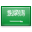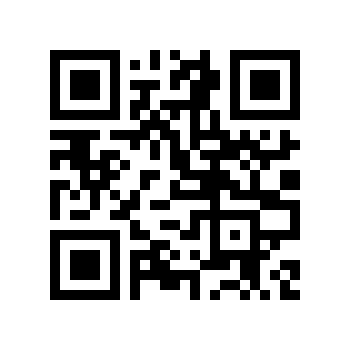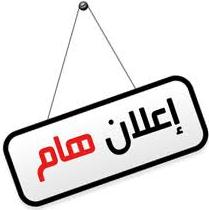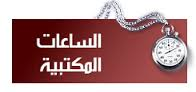# Solid of revolutionSolids of revolution (Matemateca Ime-Usp)

In mathematicsengineering, and manufacturing, a solid of revolution is a solid figure obtained by rotating a plane curve around some straight line (the axis of revolution) that lies on the same plane.

Assuming that the curve does not cross the axis, the solid's volume is equal to the length of the circle described by the figure's centroidmultiplied by the figure's area (Pappus's second centroid Theorem).

representative disk is a three-dimensional volume element of a solid of revolution. The element is created by rotating a line segment (of length w) around some axis (located r units away), so that a cylindrical volume of πr2w units is enclosed.

## Finding the volume

Two common methods for finding the volume of a solid of revolution are the disc method and the shell method of integration. To apply these methods, it is easiest to draw the graph in question; identify the area that is to be revolved about the axis of revolution; determine the volume of either a disc-shaped slice of the solid, with thickness δx, or a cylindrical shell of width δx; and then find the limiting sum of these volumes as δx approaches 0, a value which may be found by evaluating a suitable integral.

### Disk method

The disk method is used when the slice that was drawn is perpendicular to the axis of revolution; i.e. when integrating parallel to the axis of revolution.

The volume of the solid formed by rotating the area between the curves of f(x) and g(x) and the lines x = a and x = b about the x-axis is given by

${displaystyle V=pi int _{a}^{b}left|f(x)^{2}-g(x)^{2} ight|,dx,.}$If g(x) = 0 (e.g. revolving an area between the curve and the x-axis), this reduces to:

${displaystyle V=pi int _{a}^{b}f(x)^{2},dx,.}$The method can be visualized by considering a thin horizontal rectangle at y between f(y) on top and g(y) on the bottom, and revolving it about the y-axis; it forms a ring (or disc in the case that g(y) = 0), with outer radius f(y) and inner radius g(y). The area of a ring is π(R2 − r2), where R is the outer radius (in this case f(y)), and r is the inner radius (in this case g(y)). The volume of each infinitesimal disc is therefore πf(y)2 dy. The limit of the Riemann sum of the volumes of the discs between a and b becomes integral (1).

### Cylinder method

Solid of revolution demonstration
The shapes in motion, showing the solids of revolution formed by each

The cylinder method is used when the slice that was drawn is parallel to the axis of revolution; i.e. when integrating perpendicular to the axis of revolution.

The volume of the solid formed by rotating the area between the curves of f(x) and g(x) and the lines x = a and x = b about the y-axis is given by

${displaystyle V=2pi int _{a}^{b}x|f(x)-g(x)|,dx,.}$If g(x) = 0 (e.g. revolving an area between curve and y-axis), this reduces to:

${displaystyle V=2pi int _{a}^{b}x|f(x)|,dx,.}$The method can be visualized by considering a thin vertical rectangle at x with height f(x) − g(x), and revolving it about the y-axis; it forms a cylindrical shell. The lateral surface area of a cylinder is rh, where r is the radius (in this case x), and h is the height (in this case f(x) − g(x)). Summing up all of the surface areas along the interval gives the total volume.

## Parametric form

When a curve is defined by its parametric form (x(t),y(t)) in some interval [a,b], the volumes of the solids generated by revolving the curve around the x-axis or the y-axis are given by

${displaystyle V_{x}=int _{a}^{b}pi y^{2},{frac {dx}{dt}},dt,,}$${displaystyle V_{y}=int _{a}^{b}pi x^{2},{frac {dy}{dt}},dt,.}$Under the same circumstances the areas of the surfaces of the solids generated by revolving the curve around the x-axis or the y-axis are given by

${displaystyle A_{x}=int _{a}^{b}2pi y,{sqrt {left({frac {dx}{dt}} ight)^{2}+left({frac {dy}{dt}} ight)^{2}}},dt,,}$${displaystyle A_{y}=int _{a}^{b}2pi x,{sqrt {left({frac {dx}{dt}} ight)^{2}+left({frac {dy}{dt}} ight)^{2}}},dt,.}$### أهلاً ومرحباً بكم## قسم الرياضيات### التوقيت والتقويم

```_time.start(["thetimenow"]);
```

```
```

```

```

### محرك بحث جوجل```

```

### للتواصل1. الهاتف : 0164044771

تحويلة: 4771

(QR Code)

mailto:mm.mousa@mu.edu.sa### إعلانات1- الاختبار الفصلى الثانى لمقرر التحليل العددى (يوم الاحد الموافق 3 / 7/ 1440 هـ)

2- الاختبار الفصلى الثانى لمقررحساب المتجهات (يوم الثلاثاء الموافق 5 / 7 / 1440 هـ)

### الساعات المكتبيةالأثنين: 10 - 12

الثلاثاء: 8 - 10

الأربعاء: 8 - 10

### بعض الخدمات الإلكترونية لعمادة تقنية المعلومات### آلة حاسبة

```

```

### التقويم الجامعى### بعض الجوائز والتكريمات### إحصائية الموقع

عدد الصفحات: 258

البحوث والمحاضرات: 155

الزيارات: 101274